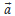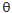# For a particle in uniform circular motion, the acceleration  at a point P(R, ) on the circle of radius R is (Here  is measured from the...

For a particle in uniform circular motion, the accelerationat a point P(R,) on the circle of radius R is (Hereis measured from the x-axis)

Anonymous User Physics Circular and Rotational Motion 12 May, 2020 60 views